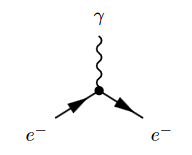# nLab electron-photon interaction

Contents

### Context

#### Fields and quanta

fields and particles in particle physics

and in the standard model of particle physics:

force field gauge bosons

scalar bosons

flavors of fundamental fermions in the
standard model of particle physics:
generation of fermions1st generation2nd generation3d generation
quarks ($q$)
up-typeup quark ($u$)charm quark ($c$)top quark ($t$)
down-typedown quark ($d$)strange quark ($s$)bottom quark ($b$)
leptons
chargedelectronmuontauon
neutralelectron neutrinomuon neutrinotau neutrino
bound states:
mesonslight mesons:
pion ($u d$)
ρ-meson ($u d$)
ω-meson ($u d$)
f1-meson
a1-meson
strange-mesons:
ϕ-meson ($s \bar s$),
kaon, K*-meson ($u s$, $d s$)
eta-meson ($u u + d d + s s$)

charmed heavy mesons:
D-meson ($u c$, $d c$, $s c$)
J/ψ-meson ($c \bar c$)
bottom heavy mesons:
B-meson ($q b$)
ϒ-meson ($b \bar b$)
baryonsnucleons:
proton $(u u d)$
neutron $(u d d)$

(also: antiparticles)

effective particles

hadrons (bound states of the above quarks)

solitons

in grand unified theory

minimally extended supersymmetric standard model

superpartners

bosinos:

dark matter candidates

Exotica

auxiliary fields

# Contents

## Idea

In quantum electrodynamics the interaction between the Dirac field $\Psi$, whose quanta are electrons, and the electromagnetic field $A$, whose quanta are photons, is encoded by the interaction Lagrangian density

$\mathbf{L}_{int} \;=\; i (\Gamma^\mu)^\alpha{}_\beta \overline{\psi}_\alpha \psi^\beta a^\mu \, dvol_\Sigma$

(with notation as as used at A first idea of quantum field theory, see this example).

For $g_{sw} \in C^\infty_{cp}(\Sigma)$ a bump function on spacetime thought of as an adiabatically switched coupling constant, the corresponding interaction action functional is the local observable

\begin{aligned} S_{int} & \coloneqq i \underset{\Sigma}{\int} g_{sw}(x) \, (\Gamma^\mu)^\alpha{}_\beta \, \overline{\mathbf{\Psi}}_\alpha(x) \cdot \mathbf{\Psi}^\beta(x) \cdot \mathbf{A}_\mu(x) \, dvol_\Sigma(x) \\ & = i \underset{\Sigma}{\int} g_{sw}(x) \, (\Gamma^\mu)^\alpha{}_\beta \, : \overline{\mathbf{\Psi}}_\alpha(x) \mathbf{\Psi}^\beta(x) \mathbf{A}_\mu(x) : \, dvol_\Sigma(x) \end{aligned} \,,

where in the first line we have the integral over a pointwise product (this def.) of three field observables (this def.), which in the second line we write equivalently as a normal ordered product, by the discusssion at Wick algebra (this def.).

(e.g. Scharf 95, (3.3.1))

The corresponding Feynman diagram isThe square of the coupling constant

$\alpha \coloneqq \tfrac{1}{4 \pi} g^2$

is called the fine structure constant.

Discussion in the context of causal perturbation theory is in